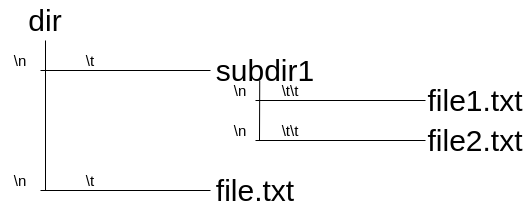# Longest Absolute File Path

Posted: 20 Mar, 2021
Difficulty: Moderate

## PROBLEM STATEMENT

#### Example:

``````For the below file system, the string S will be:
S = “dir\n\tsubdir1\n\t\tfile1.txt\n\t\tfile2.txt\n\tfile.txt”
``````#### The above string will actually be represented as:

``````dir
subdir1
file1.txt
file2.txt
file.txt
``````

#### Example:

``````file2.txt has an absolute path “dir/subdir1/file2.txt” and file.txt has path “dir/file.txt”.
The absolute file path length is the length of this string.
So absolute path length for file file2.txt will be the length of string “dir/subdir1/file2.txt” which is 21.
``````

#### You need to print the longest of all such possible absolute file path lengths.

##### Note:
``````If there is no file in the system, return 0.
``````
##### Input Format:
``````The first line of the input contains the string ‘S’.
``````

#### Note:

``````The first line of each input contains an integer that denotes the number of lines that will be given but this integer is not passed to the function because one of the aim of this question is to read input until EOF(End of the file).
``````
##### Output Format:
``````The only line of output prints a single integer denoting the longest absolute file path.
``````
##### Constraints:
``````0 <= |S| <= 5 * 10^3

Where |S| denotes the length of string S

Time limit: 1 sec
``````Approach 1

The key idea in this approach is to traverse the string line by line and count the number of tabs to get the depth of the current directory/file while maintaining a stack that stores all previous directories needed to reach the current directory/file.

Algorithm:

• Initially 'RES'=0, 'LEN'=0, which denotes the final result and the current length of the path respectively.
• Traverse through the string ‘S’ line by line.
• Count the current depth corresponding to the root directory by counting the number of tabs,i.e. Count the number of consecutive positions with S[i]==’\t’.
• If the current depth is less than or equal to stack size then pop the stack until stack size is less than the current depth, this will make sure we have the right directory order to reach the current directory/file, when we pop from the stack we reduce the ‘LEN’ variable also.
• The stack we have will store the length of the directory names which will help us in building up our answer because, in the end, all we need is the sum of lengths of the directory names.
• If currently, we have a directory we just push its name length in the stack and add it into 'LEN' variable.
• If ‘.’ is found during the process, it means currently we don’t have a directory but a file. So after adding it to the len we update our final answer.